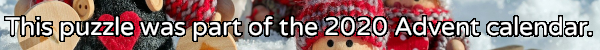mscroggs.co.uk
mscroggs.co.uksubscribe

# Puzzles

## 9 DecemberPut the digits 1 to 9 (using each digit exactly once) in the boxes so that the sums are correct. The sums should be read left to right and top to bottom ignoring the usual order of operations. For example, 4+3×2 is 14, not 10. Today's number is the product of the numbers in the red boxes.
 + × = 54 × + ÷ - ÷ = 1 ÷ - × + - = 6 =18 =6 =18
Tags: numbers, grids

## 8 DecemberThe residents of Octingham have 8 fingers. Instead of counting in base ten, they count in base eight: the digits of their numbers represent ones, eights, sixty-fours, two-hundred-and-fifty-sixes, etc instead of ones, tens, hundreds, thousands, etc.
For example, a residents of Octingham would say 12, 22 and 52 instead of our usual numbers 10, 18 and 42.
Today's number is what a resident of Octingham would call 11 squared (where the 11 is also written using the Octingham number system).

## 7 DecemberThere are 15 dominos that can be made using the numbers 0 to 4 (inclusive):
The sum of all the numbers on all these dominos is 60.
Today's number is the sum of all the numbers on all the dominos that can be made using the numbers 5 to 10 (inclusive).

## 6 DecemberThere are 12 ways of placing 2 tokens on a 2×4 grid so that no two tokens are next to each other horizonally, vertically or diagonally:
Today's number is the number of ways of placing 5 tokens on a 2×10 grid so that no two tokens are next to each other horizonally, vertically or diagonally.

## 5 DecemberCarol rolled a large handful of six-sided dice. The total of all the numbers Carol got was 521. After some calculating, Carol worked out that the probability that of her total being 521 was the same as the probability that her total being 200. How many dice did Carol roll?

## 4 DecemberToday's number is a three digit number which is equal to the sum of the cubes of its digits. One less than today's number also has this property.

## 3 DecemberPut the digits 1 to 9 (using each digit exactly once) in the boxes so that the sums are correct. The sums should be read left to right and top to bottom ignoring the usual order of operations. For example, 4+3×2 is 14, not 10. Today's number is the largest number you can make with the digits in the red boxes.
 ÷ - = 3 + + ÷ ÷ × = 1 × - + - × = 20 =91 =6 =8
Tags: numbers, grids

## 2 DecemberCarol draws a square with area 62. She then draws the smallest possible circle that this square is contained inside. Next, she draws the smallest possible square that her circle is contained inside. What is the area of her second square?

## Archive

Show me a random puzzle
▼ show ▼